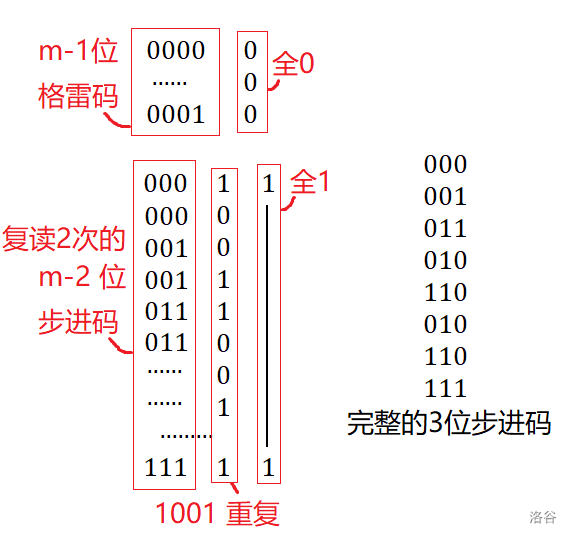command_block 的博客command_block 的博客

contest & vp 记录

posted on 2021-03-17 14:43:03 | under 记录 |

$\color{green}\bf\Delta$ 表示 contest ，$\color{blue}\bf\Delta$ 表示 vp。 $\red\bigstar$ 表示已补完。

$\color{green}\bf\Delta$ ARC 114 $\red\bigstar$

赛时

A 是个憨憨搜索。习惯了 CF 的 1A 感觉解法有点怪，所以 15min 才做掉，还丢了两发罚时。

B 是个显然的题，符合要求的集合一定是若干环的组合，而每个连通块恰有一个环，所以统计连通块数目即可。很快就做掉了。

赛后

• C Sequence Scores

$$S(i,c)=m^{n-i}\sum\limits_{j=1}^{i-1}m^{j-1}(m-c)^{i-j-1}$$

A 耗时较长，提取问题本质的过程还不够严谨迅速，对一般组合问题的分析缺乏经验和耐性。

B 性质非常顺利的看了出来，还算没掉链子。

C 体现出了发散思维上的不足，在思考时往往走错方向就一去不返。

$\color{blue}\bf\Delta$ ARC 111

赛时

B 又是个并查集题，每个连通块分别处理，树的贡献是 $n-1$ ，其他任意图的贡献都是 $n$（至少能看成一棵基环内向树）。5min 做掉了。

C 是个构造题，难度开始起来了……一开始没啥方向，发了一会呆，心一横决定随便构造些方法，至少得到些强的上界。

D 又是构造题，好像很经典的样子。

• 总结

$\color{blue}\bf\Delta$ ARC 059 $\red\bigstar$

赛前

vp 作为一种活动在机房内持续……

赛时

30min All kill

B 中字符串只有小写字母，枚举过半的字符，然后随便做。

C 当各个 $x$ 确定时就是个暴力卷积题。为一个区间时，利用乘法分配率，还是个暴力卷积题。

D 发现被删掉的按键为 0/1 是没有要求的，而显现的按键是有要求的，不妨看做每一步按键都有两种方案，最终除掉 $2^{|s|}$。

\begin{aligned} f[i]&=f[i-1]+f[i-1]\\ f[i][j]&=2*f[i-1][j-1]+f[i][j+1] \end{aligned}

$\color{blue}\bf\Delta$ ARC 107 $\red\bigstar$

赛时

30min 通过 ABCD

A 是个乘法分配律憨憨题。 B 随便化化式子。

C 看起来有点复杂，找几个性质 ：

• 两个矩阵不同当且仅当行或列上的置换不同
• 且行列交换互不干涉

D 设 $f[n][m]$ 表示 $n$ 个数和为 $m$ 的方案数，有转移 $f[n][m]=\sum\limits_{k=0}f[n-k][2(m-k)]$ 。

26min 内做了四题，感觉很稳。看 E 发现巨大神必……

• E Mex Mat

$\color{green}\bf\Delta$ ARC 115 $\red\bigstar$

赛时

60min 通过 ABCE

BC 都是憨憨构造题， B 取矩阵边界，C 考虑下界就得到答案了。

D 想了半天都不会。转而看 E ，发现是白给线段树题，于是很快做掉了。

赛后

• D Odd Degree

E 题实属出题人失误送分……看来上分一半还得靠场。

$\color{blue}\bf\Delta$ ARC 116 $\red\bigstar$

赛时

60min 通过 ABCDE

A 用约数个数定理，讨论一下 $2$ 因子个数即可。

B 排序后随便求和。

C 我的想法有点复杂，先考虑相邻两个数必须不同的情况，记 $d[x][k]$ 为长度为 $k$ 且结尾为 $x$ 的序列个数，这里 $k$ 只有 $O(\log n)$ 级别，不难利用约数求和 $O(n\log^2n)$ 求解。

D ：[SDOI2019]移动金币 的子问题。

E : [NOIP2018 提高组] 赛道修建 类似物。

F 想了一会，不会，遂知难而退。

赛后

F 属实牛逼，单独开个文章学习一下。

$\color{green}\bf\Delta$ ARC 117

赛时

10min 通过 AB

A 是憨憨构造题，随便咋搞。 B 排序后每次能操作的是一个后缀，于是差分，$+1$ 再乘起来。

赛后

• C Tricolor Pyramid

$$(-1)^{n-1}\sum\limits_{i=0}^{n-1}\binom{n-1}{i}c_i$$

• 其实赛时已经想到欧拉序乱搞了，只是怀疑正确性，没敢写。

• 总结

顺风场拼手速，逆风场练脑子。

对于真正有 ATC 风格的思维题还是不太适应，难度评分很低的题对我来说依然较为困难……

$\color{blue}\bf\Delta$ ARC 104 $\red\bigstar$

赛时

AB 是拿来凑数的吧，一个小学数学，一个暴力题。

C 有点意思，发现合法的方案每个联通块中的区间都一样长，区间 DP 即可。有若干细节。

D 分数规划后变为有负次数的 OGF ，边乘边除即可 $O(n^3k)$。中间想错了几次细节。

E 题写到一半。

$\color{blue}\bf\Delta$ AGC 031

赛时

A 各个字符只能选一个或不选，将出现次数加一乘起来。

B 发现可供操作的同色球对子中，只需要考虑中间没有同色球的那些（即相邻的那些）。记 $f[i]$ 为只触发 $\leq i$ 的操作的方案数，随便 DP 一下。

C 有点意思，若不限 $a,b$ 则是格雷码。赛后

• 总结

若做出同等难度的题目，水平较低的场中，Performance 会更高。目前来看，简单场更容易上分。

除机制外的具体原因？难的场区分度低（对于我这种蒟蒻），熟练度低导致罚时高，且卡题情况多见。

换句话说，其实是 ARC 的难度断点更加适合低水平选手罢了……

$\color{blue}\bf\Delta$ ARC 105 $\red\bigstar$

赛时

A 是迫真人肉搜索， B 瞎猜结论 gcd。

C 题搜索方案后贪心，有点麻烦，但本质不难。

D 题是个博弈，分类讨论。

• $n\bmod 2=0$

先手每次选择最大的一袋加入同一堆中（由于异或是不进位的加法，这样很难被异或掉）。

若第 $1,3,5...$ 大的和与第 $2,4,6...$ 大的和相同，则先手必败，否则必胜。

• $n\bmod 2=1$

此时后手是 $\rm Nim$ 游戏的先手。

后手只需每次将目前最大的一袋加入最大的一堆，不难发现这一堆总能大于其余的总和，故后手必胜。

E 题又是个博弈。

• $n\bmod 2=1$

合并结束时两个联通块一定一奇一偶，合并产生的影响是固定的，直接计算答案。

• $n\bmod 2=0$

若初始时与 $1,n$ 所在的两个联通块为双奇或双偶，则无法改变。

假设此时对 A 利好，若 B 改变，则 A 下一回合改回来。由于奇有偶数个，故最终不会改变。

若初始时与 $1,n$ 所在的两个联通块一奇一偶，则先手可以控制其变为双奇或双偶，于是必胜。方法类似。

$\color{blue}\bf\Delta$ ARC 112 $\red\bigstar$

赛时

60min 通过 ABCD

A 白送，B 看错题白送。此时 20min 过去了。

C 题是博弈论，显然可以划分子树为子问题。

1. $f[v]>0,e[v]=0$
2. $f[v]\leq 0,e[v]=0$
3. $e[v]=1$

D 首先观察性质，无论从哪里出发，总可以前往墙壁，然后可以转外圈，所以从外圈上出发时最劣的。

bitset 维护每个联通块覆盖的行列集合。

$\color{green}\bf\Delta$ ARC 119

赛时

A 暴力枚举 $b$ 即可。

C 先考虑如何判定某个序列 $a_{1\sim n}$ 能否被清除。

D 这个题有点阴间，过 F 的两个大佬都跳了。

• 消除整个 $S_1$ ，消除整个 $S_2$ 但饶过某一列。

• 消除整个 $S_2$ ，消除整个 $S_1$ 但饶过某一行。

E 我艹这不是憨憨题吗？

$\color{blue}\bf\Delta$ ARC 118

赛时

30min 通过 ABC

A 二分一下并线性查找。

B 先考虑 $B$ 数组能为实数的情况。先支付整数部分，然后选择剩余量较大的优先支付。

（然而被卡精度了……惨）

C 先考虑 $n=3$ 怎么做，可以构造 $\{6,10,15\}$ 。

$n$ 更大时，加入是 $6$ 或 $10$ 或 $15$ 的倍数的数。发现符合题目限制。

D 搞了半天都没搞出来，应该很接近了。这个难度 gap 有点大啊……

赛后

• D Hamiltonian Cycle

先求出 $p$ 的原（特判 $p=2$），然后将 $a,b$ 写成 $g^x,g^y$。

于是问题转化为，有一个大小为 $p-1$ 的环，每次可以前进或后退 $x$ 或 $y$ 步，要求构造一个哈密顿回路。

若 $gcd(x,y,p-1)>1$ 则无解。

求出 $d=\gcd(p-1,x)$ ，此时 $d\perp y$ ，于是 $0,y,2y,...(d-1)y$ 模 $d$ 下互不相同。

可以证明，对于 $i\in\big[0,(p-1)/d\big),j\in\big[0,d\big)$ 的 $ix+jy$ 都是互不相同的。

将上述 $(i,j)$ 列成矩阵，不难发现，题目中的操作相当于在循环矩阵中四连通移动。

记 $n=(p-1)/d,m=d$ ，则要求的是 $n\times m$ 四连通循环矩阵的哈密顿环。

由于 $nm=p-1$ 是个偶数，$n,m$ 必有一个为偶数。先横走一趟，来回绕即可构造方案。

$\color{green}\bf\Delta$ ARC 120

赛时

45min 通过 ABCD

A 题观察一下性质，讨论掉最大值就能快速算了。题出得不错。

B 题显然是 $i+j$ 相同的区域恰好被经过一次，且顺序也是定好的，故这个区域是同色的，之后就简单了。

C 题将 $A_i,B_i$ 加上 $i$ 就转化为了相邻交换构造映射最小步骤数问题。

D 题有点意思，先考虑 $A$ 只有 $0,1$ ，且 $0,1$ 个数相同的特殊情况。

E 题观察了一会，啥也没看出来。

$\color{blue}\bf\Delta$ ARC 121

赛时

A 先求出最远点对（疆域四至），然后讨论其余点对“有一个是最远点对中的点”“都不是最远点对中的点”即可。

B 若三种颜色出现次数均为偶数，则答案为 $0$ 。否则有两种颜色为奇数，两种方案 ： “两个奇数色各找一个匹配”“两个奇数色各找一个，分别找两个两个偶数色匹配”，不难排序后线性扫描。

C 猜个结论，尽量让交换减少逆序对，若无法减少，则尽量不破坏下一轮能操作的逆序对。

D 看了很久不会，于是开 E。

E 考虑容斥，钦定 $k$ 个匹配违反规则，然后树上背包即可。

F 似乎并不难，但时间不多了，遂弃。

$\color{green}\bf\Delta$ ARC 122

赛时

A 随便考虑一下贡献系数。

B 显然 $x$ 选中位数除以二。

C 经典的斐波那契拆分。

D 考虑按位贪心，若最高位为 $0,1$ 的数的数目均为偶数，则划分为两个子问题。否则找两堆数之间的最优匹配作为答案。

E 先用 Prho 分解，问题转化为有若干个二进制数，按合适的顺序排列，使得前缀 or 严格单调增加。

$\color{blue}\bf\Delta$ ARC 106 $\red\bigstar$

赛时

A 枚举憨憨题，没看清题目白给 15min+2x5min

B 随便搜一下，判两类和是否相等就好。反复确认了题面，确实是这样。一遍过了。

C 是个构造题，挺平凡的，但是因为没看清题目等种种原因 WA 了很多发。

D 用二项式拆开之后是平凡的。

E 能抽象成二分图匹配。考虑二分答案并用 Hall 定理判定。然而算错复杂度踌躇了好一会才开始写。

赛后

• [图论记录]ARC106E Medals

• [数学记录]ARC106F Figures

• 总结

这场比赛的题目较为套路，没有什么思维量，然而我却暴露出很多问题。

第一个是心态不够稳定，纯粹只是害怕，讨厌当下的境遇，又畏惧未来的麻烦。心态放松一点，发挥会平稳很多。

第二个是思考的方向不对，我总结了以下三个原则 ：

• 思路严格化。对于每一个做法，都要从复杂度，信息利用率等等角度作基本的思考，不能只是根据感觉草草判断。

迷雾中的一线光明是诱人的，大光中的一缝暗影是令人疑惧的，然而它们都只是本来的大小。

• 要把时间花在主要矛盾上。对于那些希望不大的方向，判断出阻力之后，尽早试着转向。换了好几个方向都思考不出来，也是正常的。

$\color{blue}\bf\Delta$ AGC 028

赛时

A 题 lcm 和 gcd 搞搞就没了，因为边界问题 WA 了一发。

B 题，发现贡献都是求和，没有乘积，于是可以分别考虑每两个位置之间的贡献。发现是一堆阶乘求和，推一推变成上指标求和。